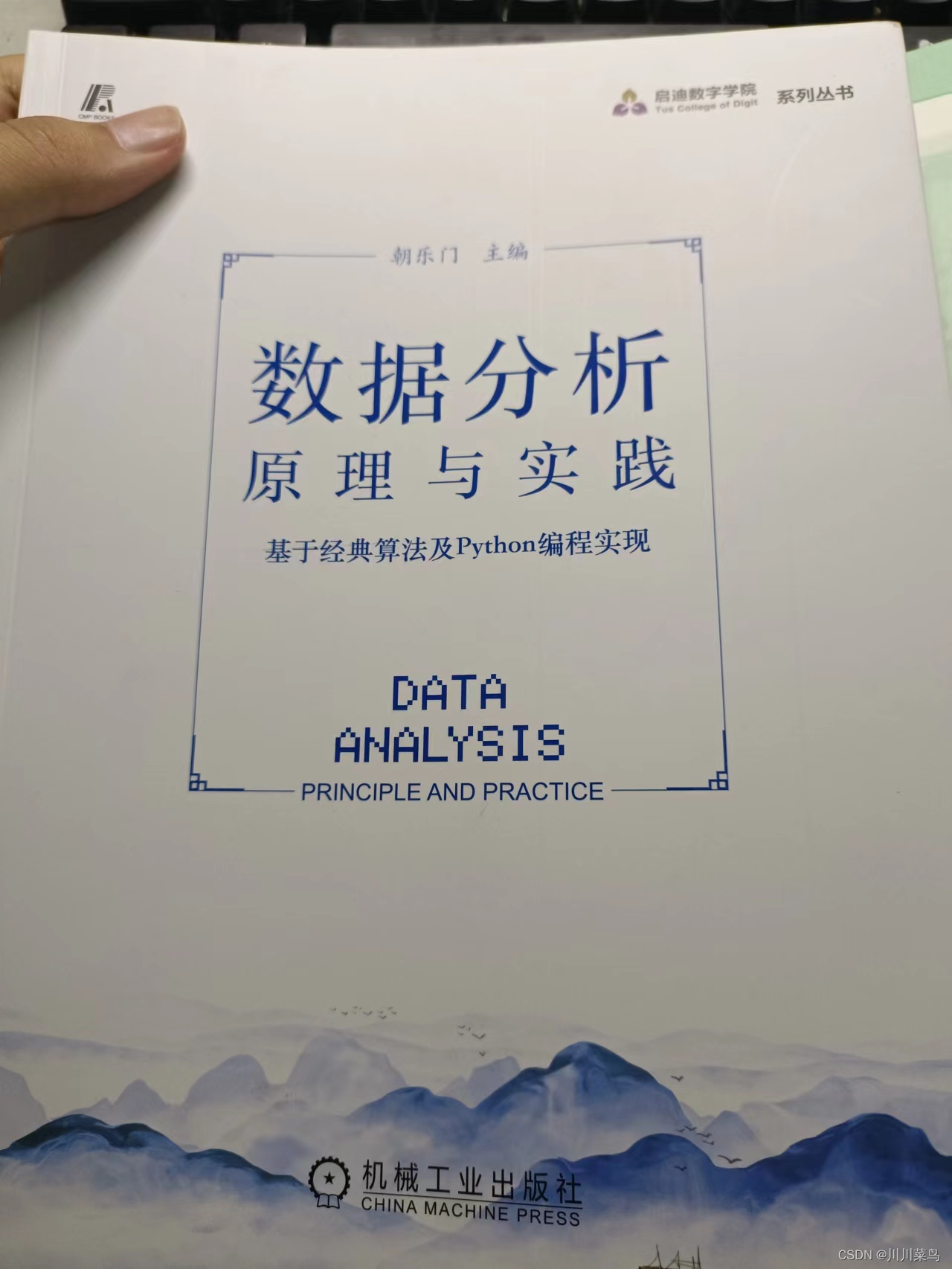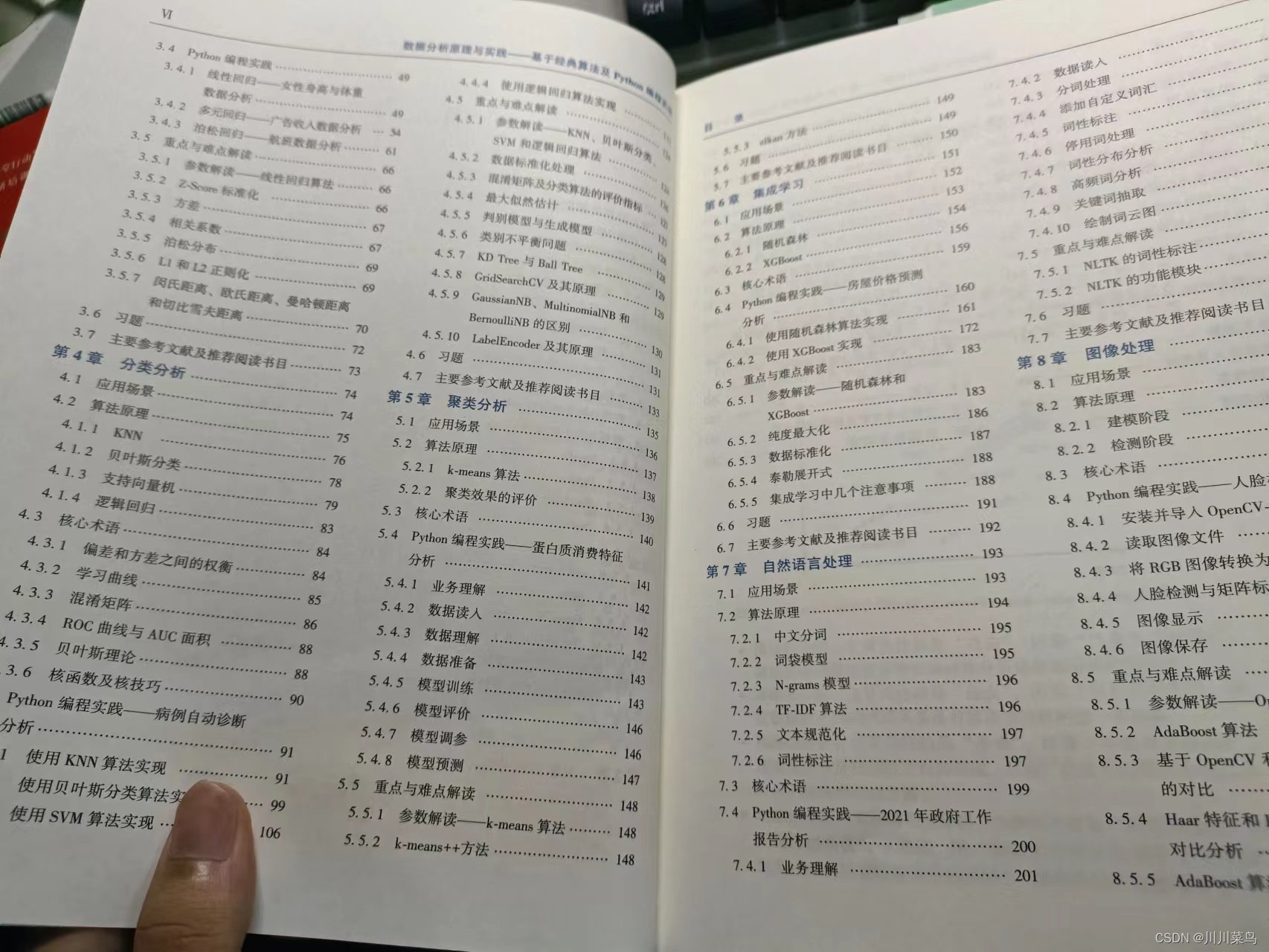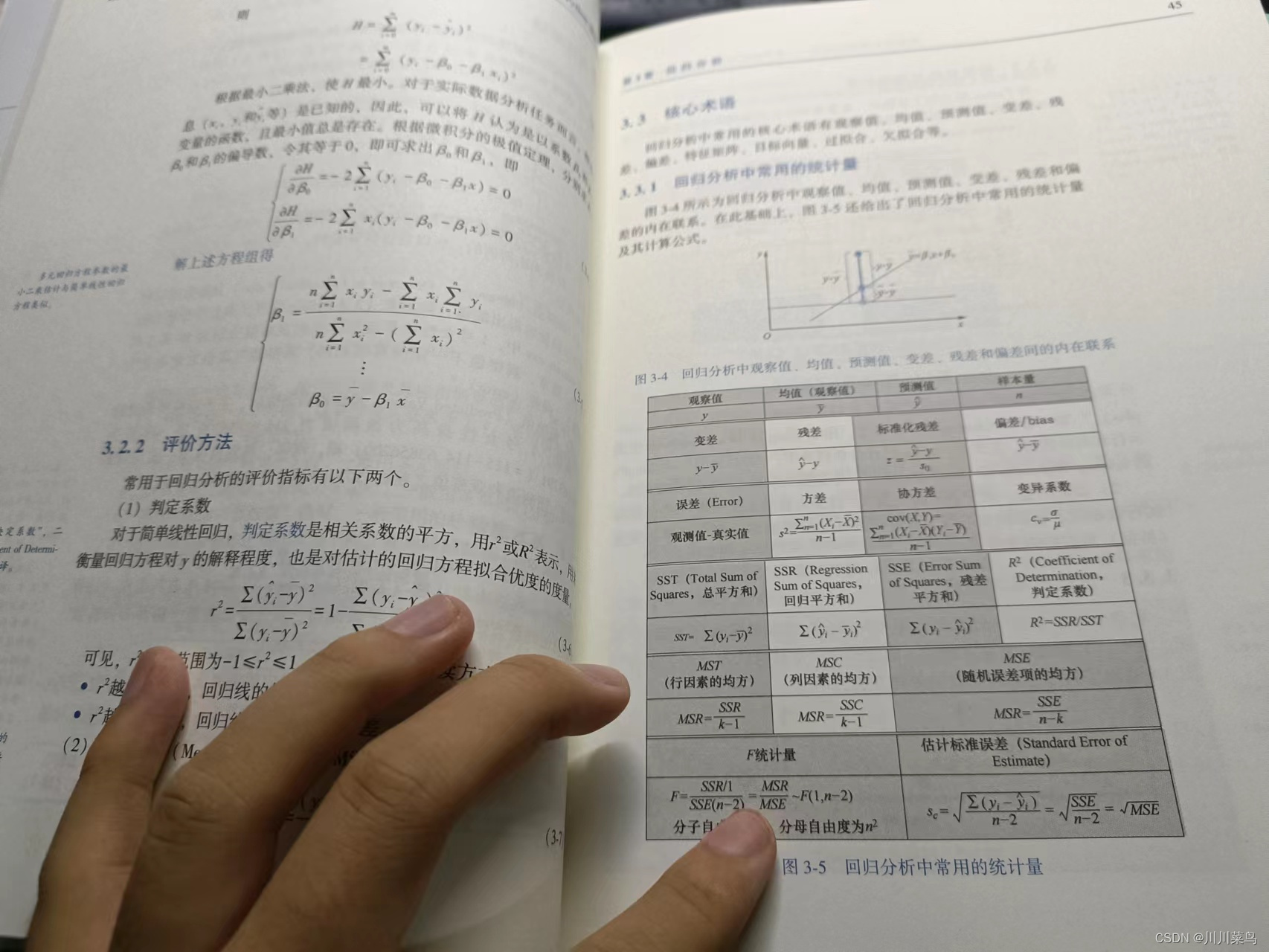### 文章目录

• 一、聚类流程与基本原理
• 二、实现层次聚类
• 2.1 导入相关库
• 2.2 读取数据
• 2.3 确定最佳集群数
• 2.4 层次聚类模型训练
• 三、本文福利

## 一、聚类流程与基本原理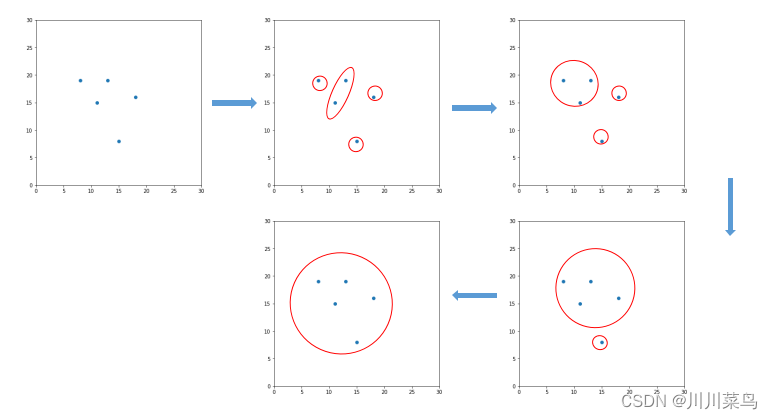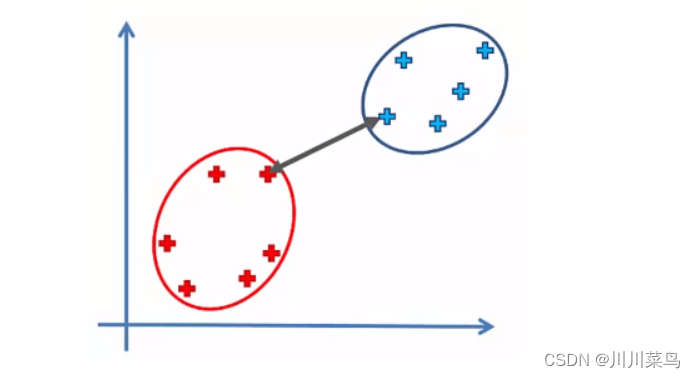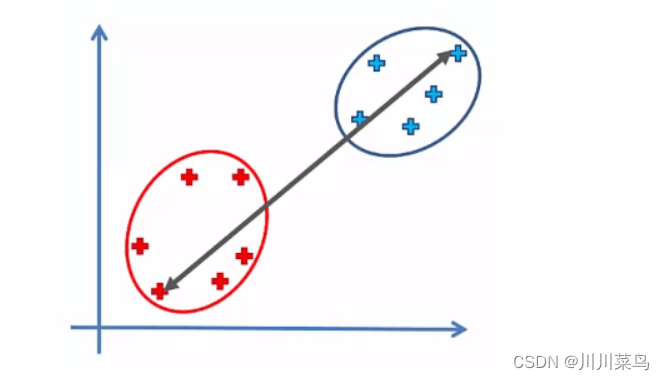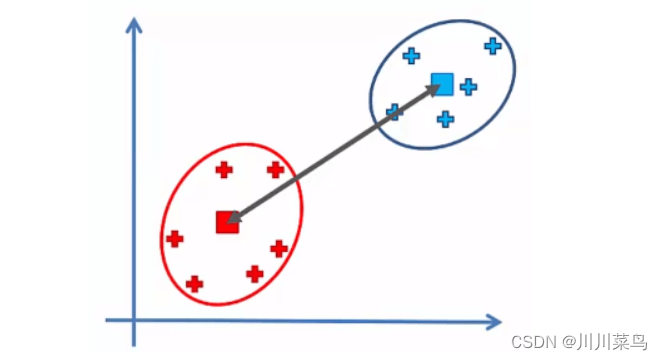## 二、实现层次聚类

``````https://wwp.lanzouv.com/i5Ru70d8yaof
``````
• 1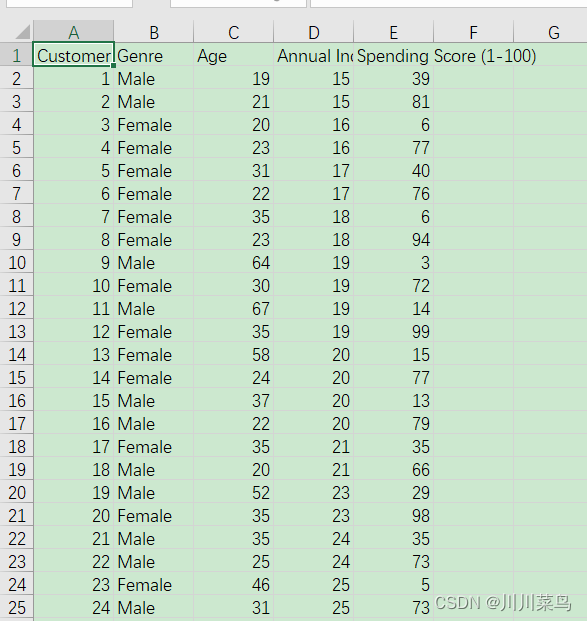### 2.1 导入相关库

``````import numpy as np
import matplotlib.pyplot as plt
import pandas as pd
``````
• 1
• 2
• 3

### 2.2 读取数据

``````ourData = pd.read_csv('Mall_Customers.csv')
``````
• 1
• 2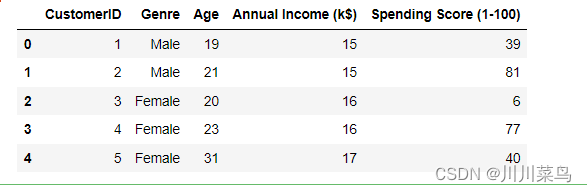``````newData = ourData.iloc[:, [3, 4]].values
newData
``````
• 1
• 2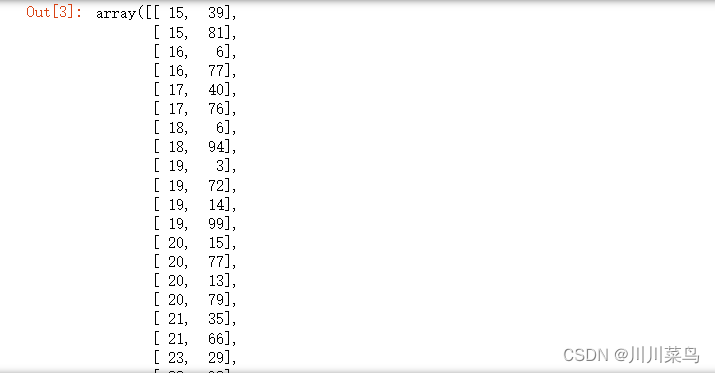### 2.3 确定最佳集群数

``````import scipy.cluster.hierarchy as sch # 导入层次聚类算法
dendrogram = sch.dendrogram(sch.linkage(newData , method = 'ward')) # 使用树状图找到最佳聚类数
plt.title('Dendrogram') # 标题
plt.xlabel('Customers') # 横标签
plt.ylabel('Euclidean distances') # 纵标签
plt.show()
``````
• 1
• 2
• 3
• 4
• 5
• 6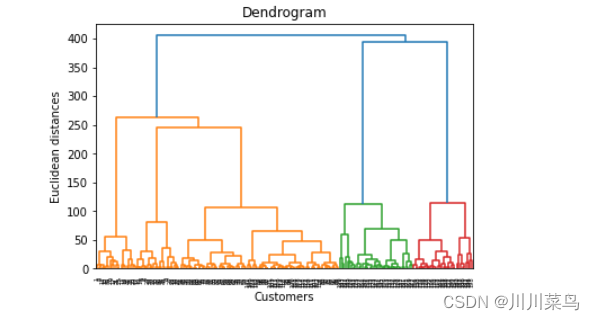### 2.4 层次聚类模型训练

``````from sklearn.cluster import AgglomerativeClustering
Agg_hc = AgglomerativeClustering(n_clusters = 5, affinity = 'euclidean', linkage = 'ward')
y_hc = Agg_hc.fit_predict(newData) # 训练数据
``````
• 1
• 2
• 3
• 4

``````plt.scatter(newData[y_hc == 0, 0], newData[y_hc == 0, 1], s = 100, c = 'red', label = 'Cluster 1') # cluster 1
plt.scatter(newData[y_hc == 1, 0], newData[y_hc == 1, 1], s = 100, c = 'blue', label = 'Cluster 2') # cluster 2
plt.scatter(newData[y_hc == 2, 0], newData[y_hc == 2, 1], s = 100, c = 'green', label = 'Cluster 3') # cluster 3
plt.scatter(newData[y_hc == 3, 0], newData[y_hc == 3, 1], s = 100, c = 'cyan', label = 'Cluster 4')  #  cluster 4
plt.scatter(newData[y_hc == 4, 0], newData[y_hc == 4, 1], s = 100, c = 'magenta', label = 'Cluster 5') #  cluster 5

plt.title('Clusters of customers')
plt.xlabel('Annual Income (k\$)')
plt.ylabel('Spending Score (1-100)')
plt.legend()
plt.show()
``````
• 1
• 2
• 3
• 4
• 5
• 6
• 7
• 8
• 9
• 10
• 11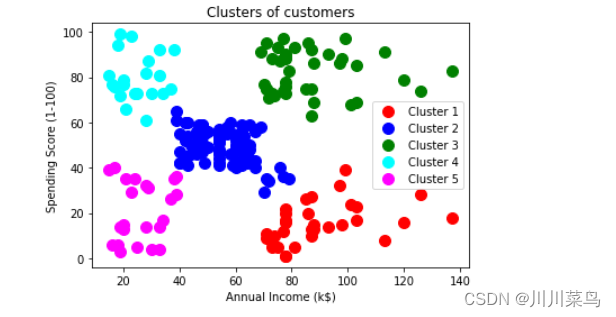## 三、本文福利